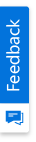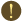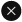We use cookies to give you the best experience on our website. If you continue to browse, then you agree to our privacy policy and cookie policy.Unfortunately, activation email could not send to your email. Please try again.# How to perform Utility functions using chart?

Platform: WinForms |
Control: Chart
Tags:

Using UtilityFunctions class we can perform Gamma, factorial, Beta and other functions used in statistical distributions formulas.

We can perform the following utility analysis in chart,

• Normal Distribution - The Normal Distribution method returns the Normal distribution for a given value.
• T Cumulative Distribution - The T Cumulative Distribution method returns the T Cumulative distribution for a given value.
• F Cumulative Distribution - The F Cumulative Distribution method returns the FCumulative distribution for a given value.

## C#

//Perform T Cumulative Distribution : Initializes the T distribution series.

series = this.chartControl1.Model.NewSeries("T Distribution",ChartSeriesType.SplineArea);

series.Text = series.Name;

//Set the degree of freedom.

n = 2;

//Calculates the Beta function.

beta = UtilityFunctions.Beta(0.5, n / 2.0);

// Calculate coefficient of T Distribution.

coef = Math.Pow(n, -0.5) / beta;

// Calculate Data Points

for (int x = -120; x <= 120; x++)

{

doubleX = x / 10.0;

y = coef / Math.Pow(1.0 + doubleX * doubleX / n, (n + 1.0) / 2.0);

//Add data points to the series.

}

//Add the series to the chart series collection.

## VB

'Perform T Cumulative Distribution : Initializes the T distribution series.

series = Me.chartControl1.Model.NewSeries("T Distribution",ChartSeriesType.SplineArea)

series.Text = series.Name

'Set the degree of freedom.

n = 2

'Calculates the Beta function.

beta = UtilityFunctions.Beta(0.5, n / 2.0)

' Calculate coefficient of T Distribution.

coef = Math.Pow(n, -0.5) / beta

' Calculate Data Points

Do

doubleX = x / 10.0

y = coef / Math.Pow(1.0 + doubleX * doubleX / n, (n + 1.0) / 2.0)

'Add data points to the series.

Loop

'Add the series to the chart series collection.

This is illustrated in the Chart UG in this topic:

Utility Formulas

2X faster development

The ultimate WinForms UI toolkit to boost your development speed.You are using an outdated version of Internet Explorer that may not display all features of this and other websites. Upgrade to Internet Explorer 8 or newer for a better experience.